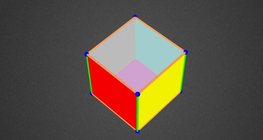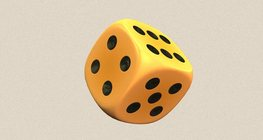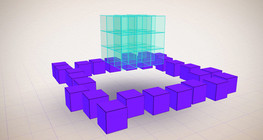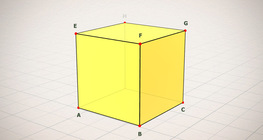Quantity: 0

Total: 0,00

# Nets of a cuboid (exercises)

### Nets of a cuboid (exercises)

This animation demonstrates the different nets of a cuboid and includes a game.

Mathematics

Keywords

net of a cuboid, net, cuboid, solids, exercise, geometry, mathematics

### Related items

Information Cuboid#### Cuboid

A cuboid is a polyhedron with six rectangular faces.

Information Grouping of cuboids#### Grouping of cuboids

This animation demonstrates various types of cuboids through everyday objects.

Information Net of a cube (exercises)#### Net of a cube (exercises)

Not all nets consisting of 6 congruent squares are foldable into cubes.

Information Colouring a cube#### Colouring a cube

Colouring the vertices, edges and faces of a given cube according to the criteria specified in the exercise.

Information Cube#### Cube

This animation demonstrates the components (vertices, edges, diagonals and faces) of the cube, one of the Platonic solids.

Information Cube of cubes#### Cube of cubes

An exercise about the regular hexahedron built from unit cubes to help deepen your knowledge of cubes.

Information Dice#### Dice

Regular dice can be used for solving statistical and probability exercises.

Information Notable products#### Notable products

A spectacular demonstration of algebraic expressions.

Information Shape games#### Shape games

Find views and nets of three-dimensional shapes.

Information Volume and surface area (exercise)#### Volume and surface area (exercise)

An exercise about the volume and surface area of solids generated from a ´base cube´.

Information Constructing shapes (3D)#### Constructing shapes (3D)

Building 3D shapes into a given cube mesh with the help of several views.

Information Cube (exercises)#### Cube (exercises)

Edges, diagonals and faces of a cube can be identified by its vertices.

Information Cube puzzle#### Cube puzzle

Building cubes shown in several views from the available unit cubes aids spatial vision and other skills.

Information Cube sections (exercise)#### Cube sections (exercise)

Examining solids formed by the intersection of a cube and a plane.

Information Building shapes (multi colour)#### Building shapes (multi colour)

Build 3D shapes from unit cubes with the help of several views.

Information Building shapes (one colour)#### Building shapes (one colour)

Build 3D shapes from unit cubes with the help of several views.